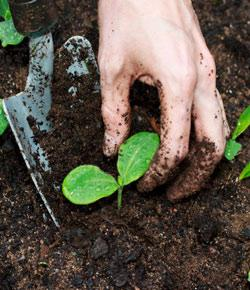# Soil Science 1

30 Questions | Total Attempts: 2567SettingsA basic quiz covering the first few chapters of a soil science textbook.

Related Topics
• 1.
Although subsoil is more difficult to obtain, it is generally equally as good as topsoil for landscaping purposes.
• A.

True

• B.

False

• 2.
Which of the following pH values represents a neutral condition?
• A.

5.0

• B.

1.0

• C.

6.0

• D.

10

• E.

7.0

• 3.
The lithosphere is made up of ____________.
• A.

Air

• B.

Rock

• C.

Plants and animals

• D.

Water

• E.

All of the above

• 4.
Which statement is false about the ability we have to change the soil?
• A.

Mineral make up is relatively unchangeable.

• B.

Soil texture is relatively changeable.

• C.

Soil structure is relatively changeable.

• D.

Organic matter content is relatively changeable.

• 5.
Adsorption is the attraction of ions to the surface of particles.
• A.

True

• B.

False

• 6.
Most (usually 80% or more) of soil potassium and calcium can be found in the form of __________.
• A.

Structural components of minerals

• B.

Exchangeable ions

• C.

Organic compounds

• D.

Dissolved substances

• 7.
In a load of 10 cubic meters of topsoil, approximately how many cubic meters of the volume would be solid material?
• A.

1

• B.

2.5

• C.

4

• D.

5

• E.

7.5

• 8.
Compared to silt, clay-sized soil particles are characterized by _______.
• A.

Greater proportion of primary minerals

• B.

Greater attraction for water

• C.

Less tendency to form hard clods when dry

• D.

Less capacity to hold nutrients in plant-available forms

• 9.
The water in the soil typically differs from pure water because the soil water _________.
• A.

Is restrained in its flow by attraction to particle surfaces

• B.

Contains organic compounds

• C.

Contains mineral nutrients

• D.

All of the above

• E.

None of the above

• 10.
Increasing the organic matter content of a soil is likely to ___________.
• A.

Increase the soil's water holding capacity

• B.

Decrease the soil's water holding capacity

• C.

Have no effect on water holding capacity

• 11.
• A.

Pore space

• B.

Texture

• C.

Structure

• D.

Solution

• E.

Profile

• 12.
Solum occupies the ____________ part of the regolith.
• A.

Lower

• B.

Upper

• C.

Younger

• D.

Both B and C

• 13.
Which of the following is considered to be a plant macronutrient?
• A.

Ca

• B.

N

• C.

P

• D.

S

• E.

All of the above

• 14.
A, B, C, and E horizons can be found in any true soil.
• A.

True

• B.

False

• 15.
Which statement of false about soil air in comparison to atmospheric air?
• A.

Soil air is homogeneous throughout a large region.

• B.

The oxygen content is lower.

• C.

The carbon dioxide content is much higher.

• D.

Soil air has more moisture.

• 16.
Which of the following is an essential plant nutrient?
• A.

Al

• B.

Cu

• C.

Pb

• D.

Sr

• E.

Yr

• 17.
Subsoil is generally equivalent to which soil horizon?
• A.

A

• B.

B

• C.

C

• D.

O

• E.

R

• 18.
A soil profile consists of _____________.
• A.

The set of layers seen in a vertical cross section of a soil

• B.

The sum of chemical and physical data known about a soil

• C.

The boundaries of a particular soil

• D.

The way a soil "feels"

• E.

The general outline of a soil or group of soils when viewed from the side

• 19.
Which of the following pH values represents the most acid condition?
• A.

1.0

• B.

5.55

• C.

7.0

• D.

10

• E.

100

• 20.
Hydroxyl ion concentrations are greatest in a soil solution with a pH value of ______.
• A.

0.1

• B.

4.0

• C.

5.0

• D.

6.5

• 21.
Gram per gram, which material can hold more nutrients for plants?
• A.

Clay

• B.

Humus

• C.

Saprolite

• D.

Silt

• 22.
In a given soil, the horizon with the highest organic matter content is generally the ___ horizon.
• A.

A

• B.

B

• C.

C

• D.

E

• E.

R

• 23.
Which soil-forming process is most repsonsible for the development of B horizons?
• A.

• B.

Losses

• C.

Transformations

• D.

Translocations

• 24.
Alluvial parent materials are those that have been laid down in former lake bottoms.
• A.

True

• B.

False

• 25.
Silicate clay accumulation is most common in the ___ horizon?
• A.

A

• B.

B

• C.

C

• D.

E

• E.

O

• F.

R

• 26.
Talus slopes and detritus are good examples of which?
• A.

Secondary and tertiary minerals

• B.

Sedimentary rocks

• C.

Colluvium

• D.

• E.

Rhyolite

• 27.
The steep, convex portion of a hillslope is called the ____________.
• A.

Backslope

• B.

Summit

• C.

Shoulder

• D.

Toeslope

• 28.
A soil developed in transported parent materials will likely have properties related to the properties of the rock below the C horizon.
• A.

True

• B.

False

• 29.
Physical weathering ___________________.
• A.

Leads to the formation of clay mineral particles

• B.

Increases the surface area of soil minerals

• C.

Releases essential plant nutrients

• D.

Is the result of water mixing with the carbon dioxide respired by plants and microorganisms

• 30.
Alluvium typically results in well developed soil.
• A.

True

• B.

False